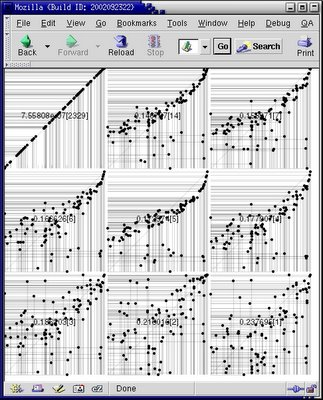## 29 January 2006

### Past projects (IV)

One day I found a book about 'genetic programming' in a colleague's office and I wondered if I could use this (the answer was no) to discover a mathematical way how to describe the signal from BAC clones spotted on a CGH array. Note that 'genetic programming' is different from 'genetic algorithms': in the first one there is a mathematical function which evolve whereas this is a popolulation of data in the second one. See http://www.geneticprogramming.com/Tutorial/ for a tutorial about Genetic Programming.

So I wrote a C++ program that took as input a table of result, a list of expected number and I trained a mathematical function to evolve with those data:This SVG figure shows nine steps of the evolution process. In each setp, on the X axis are displayed the expected values and the experimental values (calculated from the evolving methematical function) are on the Y axis. The program stopped when a defined fitness was reached. Fun to write.